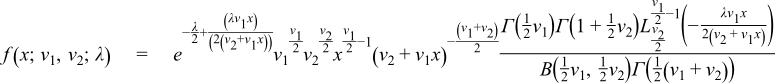#Boost C++ Libraries

...one of the most highly regarded and expertly designed C++ library projects in the world.

#### Noncentral F Distribution

`#include <boost/math/distributions/non_central_f.hpp>`
```namespace boost{ namespace math{

template <class RealType = double,
class Policy   = policies::policy<> >
class non_central_f_distribution;

typedef non_central_f_distribution<> non_central_f;

template <class RealType, class Policy>
class non_central_f_distribution
{
public:
typedef RealType  value_type;
typedef Policy    policy_type;

// Constructor:
non_central_f_distribution(RealType v1, RealType v2, RealType lambda);

// Accessor to degrees_of_freedom parameters v1 & v2:
RealType degrees_of_freedom1()const;
RealType degrees_of_freedom2()const;

// Accessor to non-centrality parameter lambda:
RealType non_centrality()const;
};

}} // namespaces
```

The noncentral F distribution is a generalization of the Fisher F Distribution. It is defined as the ratio

```F = (X/v1) / (Y/v2)
```

where X is a noncentral χ2 random variable with v1 degrees of freedom and non-centrality parameter λ, and Y is a central χ2 random variable with v2 degrees of freedom.

This gives the following PDF:where Lab(c) is a generalised Laguerre polynomial and B(a,b) is the beta function, orThe following graph illustrates how the distribution changes for different values of λ:##### Member Functions
```non_central_f_distribution(RealType v1, RealType v2, RealType lambda);
```

Constructs a non-central beta distribution with parameters v1 and v2 and non-centrality parameter lambda.

Requires v1 > 0, v2 > 0 and lambda >= 0, otherwise calls domain_error.

```RealType degrees_of_freedom1()const;
```

Returns the parameter v1 from which this object was constructed.

```RealType degrees_of_freedom2()const;
```

Returns the parameter v2 from which this object was constructed.

```RealType non_centrality()const;
```

Returns the non-centrality parameter lambda from which this object was constructed.

##### Non-member Accessors

All the usual non-member accessor functions that are generic to all distributions are supported: Cumulative Distribution Function, Probability Density Function, Quantile, Hazard Function, Cumulative Hazard Function, mean, median, mode, variance, standard deviation, skewness, kurtosis, kurtosis_excess, range and support.

The domain of the random variable is [0, +∞].

##### Accuracy

This distribution is implemented in terms of the Noncentral Beta Distribution: refer to that distribution for accuracy data.

##### Tests

Since this distribution is implemented by adapting another distribution, the tests consist of basic sanity checks computed by the R-2.5.1 Math library statistical package and its pbeta and dbeta functions.

##### Implementation

In the following table v1 and v2 are the first and second degrees of freedom parameters of the distribution, λ is the non-centrality parameter, x is the random variate, p is the probability, and q = 1-p.

Function

Implementation Notes

pdf

Implemented in terms of the non-central beta PDF using the relation:

f(x;v1,v2;λ) = (v1/v2) / ((1+y)*(1+y)) * g(y/(1+y);v1/2,v2/2;λ)

where g(x; a, b; λ) is the non central beta PDF, and:

y = x * v1 / v2

cdf

Using the relation:

p = By(v1/2, v2/2; λ)

where Bx(a, b; λ) is the noncentral beta distribution CDF and

y = x * v1 / v2

cdf complement

Using the relation:

q = 1 - By(v1/2, v2/2; λ)

where 1 - Bx(a, b; λ) is the complement of the noncentral beta distribution CDF and

y = x * v1 / v2

quantile

Using the relation:

x = (bx / (1-bx)) * (v1 / v2)

where

bx = Qp-1(v1/2, v2/2; λ)

and

Qp-1(v1/2, v2/2; λ)

is the noncentral beta quantile.

quantile

from the complement

Using the relation:

x = (bx / (1-bx)) * (v1 / v2)

where

bx = QCq-1(v1/2, v2/2; λ)

and

QCq-1(v1/2, v2/2; λ)

is the noncentral beta quantile from the complement.

mean

v2 * (v1 + l) / (v1 * (v2 - 2))

mode

By numeric maximalisation of the PDF.

variance

skewness

kurtosis and kurtosis excess

Some analytic properties of noncentral distributions (particularly unimodality, and monotonicity of their modes) are surveyed and summarized by:

Andrea van Aubel & Wolfgang Gawronski, Applied Mathematics and Computation, 141 (2003) 3-12.

 Copyright © 2006-2010, 2012, 2013 Paul A. Bristow, Christopher Kormanyos, Hubert Holin, Bruno Lalande, John Maddock, Johan Råde, Gautam Sewani, Benjamin Sobotta, Thijs van den Berg, Daryle Walker and Xiaogang Zhang Distributed under the Boost Software License, Version 1.0. (See accompanying file LICENSE_1_0.txt or copy at http://www.boost.org/LICENSE_1_0.txt)